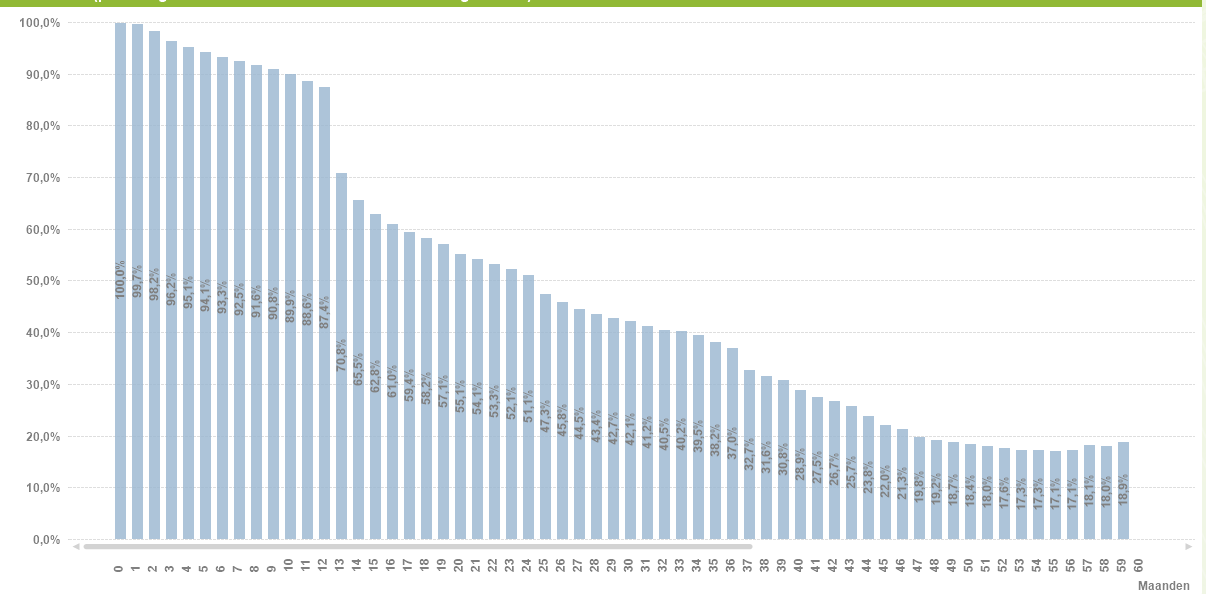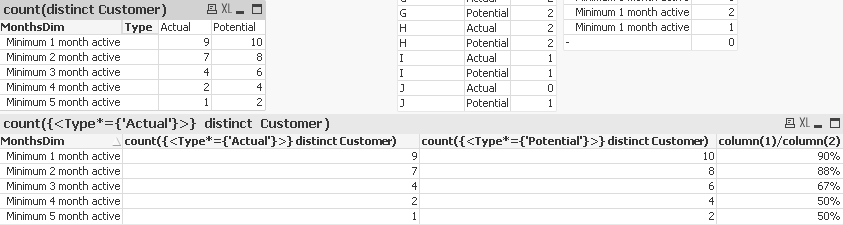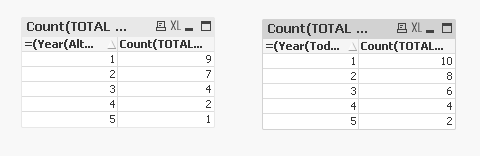QlikView App Development

Discussion Board for collaboration related to QlikView App Development.

New Contributor III

How to calculate the expression for every possible value of the dimension in a chart?

Hi all,

I'm trying to create a chart where the expression is calculated separately for every value of the chart's dimension.

Dimension: DimMonths (ranging from 1 to 100, generated with AutoGenerate)

Expression: Count( {< ActiveMonths = {">=\$(=DimMonths)"} >} Customer) / Count( {< PotentialMonths = {">=\$(=DimMonths)"} >} Customer)

End result should look like this:The above expression obviously doesn't work, because Qlikview does not calculate the expression for all possible values of DimMonths. I made it work with an nested IF-statement, but with 100+ distinct values this is obviously terrible for performance, readability and maintenance:

IF(DimMonths = 0,COUNT( {< ActiveMonths = {">=0"} >} Customer) / COUNT( {< PotentialMonths = {">=0"} >} Customer),

IF(DimMonths = 1,COUNT( {< ActiveMonths = {">=1"} >} Customer) / COUNT( {< PotentialMonths = {">=1"} >} Customer),

IF(DimMonths = 2,COUNT( {< ActiveMonths = {">=2"} >} Customer) / COUNT( {< PotentialMonths = {">=2"} >} Customer),

Etc...

Etc...

Does anyone know a better solution?

-----------------------------------------------------------------

Here is some (simplified) sample data:

DimMonths:

AUTOGENERATE(5);

CustomerContractStartContractEndPotentialMonths
(= Nov16– ContractStart)
ActiveMonths
(= ISNULL(ContractEnd,Nov16) – ContractStart)
AJune16-55
BJune16Sep1653
CJuli16Okt1643
DJuli16-44
EAug16Sep1631
FAug16Okt1632
GSep16-22
HSep16-22
IOkt16-11
JOkt16Okt1610

I'm looking for an expression that gives me this result. I cannot make the calculations in the load script, because the graph needs to be responsive to other dimensions.

ActivePotentialRest
Minimal 1 month active91090%
Minimal 2 month active7888%
Minimal 3 month active4666%
Minimal 4 month active2450%
Minimal 5 month active1250%
1 Solution

Accepted SolutionsMVP

Re: How to calculate the expression for every possible value of the dimension in a chart?

I would do it almost exactly like Jakub suggests, but instead of converting my main table, I'd have the table he shows be an additional table, because I figure we still want to track contract start and end dates, and I don't want those repeated on the new table.

See attached.

Months:
CROSSTABLE (Type,Months)
Customer
,PotentialMonths as Potential
,ActualMonths as Actual
RESIDENT Customers
;

AsOf:
,dual('Minimum ' & (Months+1-iterno()) & ' month active',Months+1-iterno()) as MonthsDim
WHILE Months+1-iterno() > 0
;
WHILE iterno() <= Max
;
AUTOGENERATE fieldvaluecount
('Months')
;13 RepliesMVP

Re: How to calculate the expression for every possible value of the dimension in a chart?

Would you be able to share a sample?

Valued Contributor III

Re: How to calculate the expression for every possible value of the dimension in a chart?

Count( {< ActiveMonths >= {"DimMonths"} >} Customer) / Count( {< PotentialMonths >= {"DimMonths"} >} Customer)

--> this is wrong I think

you could try this:

Count( {< ActiveMonths = {">=\$(=only(DimMonths))"} >} Customer) / Count( {< PotentialMonths = {">=\$(=only(DimMonths))"} >} Customer)

New Contributor III

Re: How to calculate the expression for every possible value of the dimension in a chart?

Hi Sunny T, i added some (simplified) sample data to the original post

New Contributor III

Re: How to calculate the expression for every possible value of the dimension in a chart?

Hi Robin, thanks for your suggestion!

Obviously my expression had incorrect syntax, i changed that in my original post. The problem with this solution is that DimMonths only works in set analysis when it is a unique value or a variable. But because DimMonths is my dimension, it is different for every row and the expression should be recalculated for every row with a different value of DimMonths. Set analysis doesn't do that (i'm not sure, but that's what i read on this community), so I'm looking for a way around it...MVP

Re: How to calculate the expression for every possible value of the dimension in a chart?

I am able to get the two numbers in two separate charts, but having a hard time putting them together in one chart. May be somebody else have a better idea....New Contributor III

Re: How to calculate the expression for every possible value of the dimension in a chart?

Im trying this solution now. It is complex but it works, however performance isn't any better than a nested-if solution (with my 60 months x 500.000 rows)

Evaluating "sets" in the context of a dimensionMVP

Re: How to calculate the expression for every possible value of the dimension in a chart?

Let us know if you find a solution. I would be eager to know if there is a way to do this. Also, we might be able to seek the expert's help if you run into any issue johnw

Best,

Sunny

HighlightedPartner

Re: How to calculate the expression for every possible value of the dimension in a chart?

You could solve it with some data modelling, I think.

Consider having your main table in this form:

CustomerMonthsPotentialFlag
A5

Potential

A4Active
B1Potential
B1Active
C2Potential
C0Active
.........

Then, have another table in this form ("100" is a placeholder for what the maximum number in Months column is - it's easy enough to get it after you load all the data in the above table, and generate the whole thing below automatically with Autogenerate and While):

MonthsDimMonths
100100
99100
9999
98100
9899
9898
......

(it's kinda like a table that would connect months to YTD months, only in reverse)

With such a data model, use MonthsDim as dimension, and your formulas will become pretty simple:

COUNT( {< PotentialFlag = {'Active'} >} Customer) / COUNT( {< PotentialFlag = {'Potential'} >} Customer)

This is not precalculating anything - just linking data differently - so selections will keep working.

Of course, whether you can use this suggestion or not depends on the rest of your data model. But maybe you can adapt itMVP

Re: How to calculate the expression for every possible value of the dimension in a chart?

I would do it almost exactly like Jakub suggests, but instead of converting my main table, I'd have the table he shows be an additional table, because I figure we still want to track contract start and end dates, and I don't want those repeated on the new table.

See attached.

Months:
CROSSTABLE (Type,Months)
Customer
,PotentialMonths as Potential
,ActualMonths as Actual
RESIDENT Customers
;

AsOf: